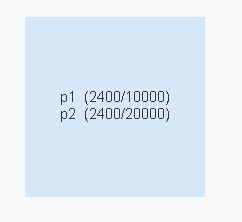# QlikView App Development

Discussion Board for collaboration related to QlikView App Development.

Announcements
Modernize Your QlikView Deployment webinar, Nov. 3rd. REGISTER
cancel
Showing results for
Did you mean:
HighlightedContributor III

## obtained / total values in textbox

I have data in sheet like this

 products obtained total p1 1200 10000 p2 1000 20000 p3 200 30000 p1 1200 10000 p2 1400 20000 p3 600 30000

here if i get sum of products then p1 shows 2400 p2=2400 and p3=800 .. so here i get 2400 top value against twp products ..

so i want to get top values in textbox and want like this in textbox

p1 (2400/10000)  p2 (2400/20000)

how i do this ?

1 Solution

Accepted Solutions
HighlightedMVP

Use this expression in text box..

=Concat({<products = {"=Rank(SUM(obtained))<=2"}>}DISTINCT Aggr(products & '  ' & '(' & SUM(obtained) & '/' & SUM(Distinct total) & ')',products),CHR(10))This is the output.

19 Replies
HighlightedMVP

It should be something like this:

='p1 (' & Sum({<products={'p1'}>} amount) & '/' & Sum(TOTAL Sum({<products={'p1'}>} amount) & ') p2 (' & Sum(Sum({<products={'p3'}>} amount) & '/' & Sum(TOTAL {<products={'p3'}>} amount) & ')'

HighlightedContributor III

but here i dont want to hard code p1, p2 .. i want to get through column name

and also this shows an brackets error and nested aggregation not allowed

HighlightedMVP

How p1 2400?

How p2 2400?

Also when you are showing TOTAL then how this total coming?

Are p1, p2, p3 have any other common field or parent field?

HighlightedContributor III

because p1 has 2 values 1200 and 1200=2400 and

p2 has 1000 and 1400 =2400

and yes there is another sheet in excel which has common filed products

check attached sheet

HighlightedContributor III

any update

HighlightedMVP

Use this expression in text box..

=Concat({<products = {"=Rank(SUM(obtained))<=2"}>}DISTINCT Aggr(products & '  ' & '(' & SUM(obtained) & '/' & SUM(Distinct total) & ')',products),CHR(10))This is the output.

HighlightedMVP

It should be more like:

='p1 (' & Sum({<products={'p1'}>} amount) & '/' & Sum(TOTAL {<products={'p1'}>} amount) & ') p2 (' & Sum{<products={'p3'}>} amount) & '/' & Sum(TOTAL {<products={'p3'}>} amount) & ')'

HighlightedContributor IIIwhy this shows red line?

HighlightedMVP

Don't worry about red lines.. Just check at the top left expression OK is there or not..